# Input and Output in Python

P N Ramesh

June 2, 2020, 2:38 p.m. | Python Tutorial

## Input and Output in Python - Part I (Output and Formatting)

Programming learning is not about learning a programming language's syntax. If you thoroughly read any materials, such as books or tutorials, then programming is not meant to be learned. Programming is all about constructing logic, syntax only plays a 5 percent role.

If you are good in the building of logic then in just a few days you can learn any programming language. My posts will concentrate on both constructing logic and mapping the syntax to the logic created.

Programming is a series of statements written to perform any function on a computer, two essential operations in any function are called input and output display. Typically we learn programming with a console application which is an application based on text. In console application inputs get from keyboard and outputs displayed to the monitor. The figure below indicates Console Use.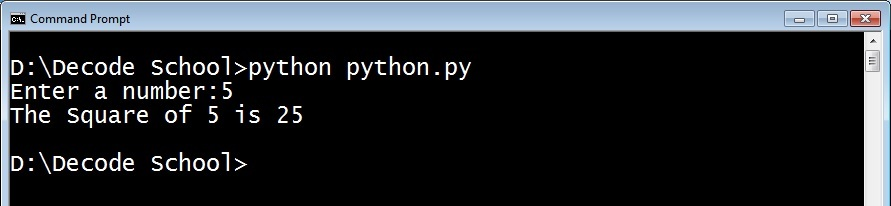Inputs and outputs are available in different formats such as series, numbers, whole numbers and decimal numbers etc. In this review, we dive deep into the dynamics of Input and Output Statements.

### Example 1: Printing the "Hello World"

``print("Hello world")``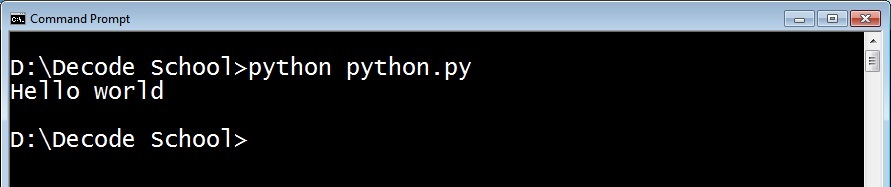The above statement or command shows the "Hello World" string in the console. Yeah, printing is the function statement used to display something in the console.

### Example 2 : Simple Input and Output

When you want a string to be entered by the user and the machine to display the same.

``text = input("Enter your Name:")   print(text)``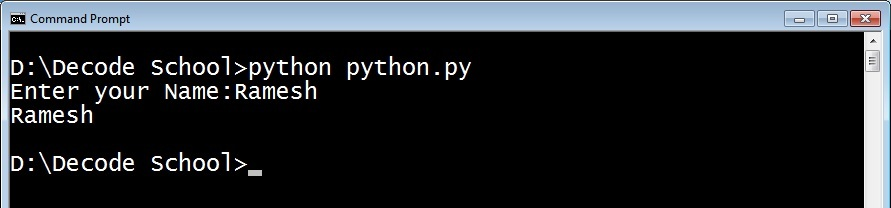The first line shows the "Enter Your Name" message and reads the user's input and saves the memory, the location of the memory being referenced by the name (variable) named text. The second statement prints the string referenced by the name text.

You will remember that in Example 1, Hello World is prefixed and suffixed with double quotes inside the print statement, but in Example 2 text is not protected by double quotes, since we want to print content inside text and not text.

Yeah, in this learning process we concentrate intensely on output statements and subsequent input statements for a few miles.

### print Statement:

How to print a varible value with additional pharase, such as "Entered Name is:"? We can do that in many ways.

### Example 3:

First option is we can use string concatenation like:

``name = input("Enter your Name:")print("Entered Name is: " + name)``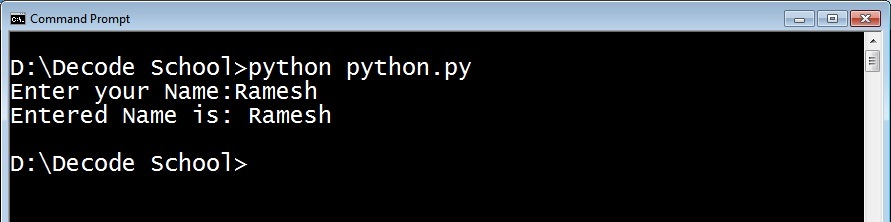This approach generates an exception if the variable has numbers (integer or float)

### Example 4:

second option is you can pass the pharase and varible as two arguments to print funtion

``````name = input("Enter your Name:")print("Entered Name is: ", name)
``````

However, formatted print is suitable to print outputs in different formats.

### Example 5:

``````name = input("Enter your Name:")print("Entered Name is: {} ".format(name))
``````

The above example shows the basic formatting, and the following examples show different formatted printing scopes.

### Example 6:

``fname = input("Enter First Name:") lname = input("Enter Second Name:")print("First Name: {} \nLast Name: {}".format(fname,lname))``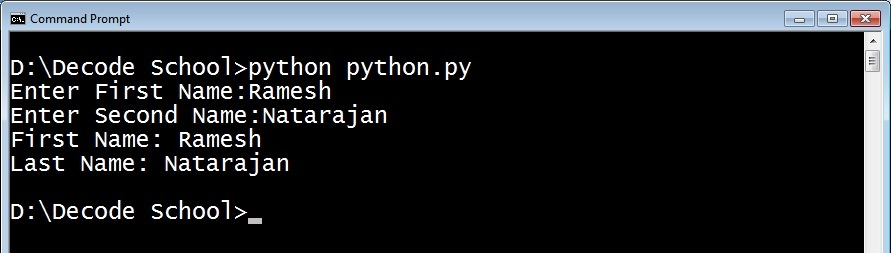two {} (set brackets) are reserved for the value of variables fname and lname.

\n prints the new line in the console. using this format function, we could do right and center alignment the text in the specified length

Like \n there are several other escape sequences, the follwing table illustrates the same.

### Example 7:

``````print("Hello\tRamesh")                 #             \t leaves a tab space
print("Hello \' Ramesh")                #             \' prints single quotes( ' )
print("Hello \" Ramesh")                #             \" prints double quotes( " )
print("Hello \\ Ramesh")                #             \\ prints backslash ( \ )
print("Hello\bRamesh")                  #             \b backspace (it deletes 'o')
print("Hello and welcome\rRamesh")      #             \r carriage return, cursor moves to                                                                                                                          starting position of line
print("Hello\vRamesh")  		#             \v Vertical Space
``````

### Example 8:

``````print("{:>10}".format("Ramesh"))             #Right Alignment
print("{:^10}".format("Ramesh"))             #Center Alignment
print("{:10}".format("Ramesh"))               #left Alignment
print('{:_<10}'.format('Ramesh'))             #left alignment and fill
``````

### Example 9:

we can truncate string using .

``print('{:.10}'.format('Hello Everyone'))``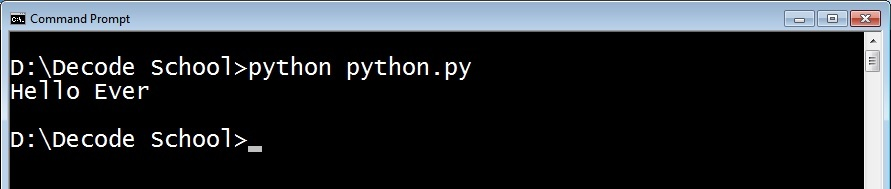First 10 characters will be printed in the large string.

Few more applications using String Formatting in Print

### Example 10:

``````
print("1 X 9 = {:>2}".format('5'))print("2 X 9 = {:>2}".format('10'))print("3 X 9 = {:>2}".format('15'))print("4 X 9 = {:>2}".format('20'))print("5 X 9 = {:>2}".format('25'))``````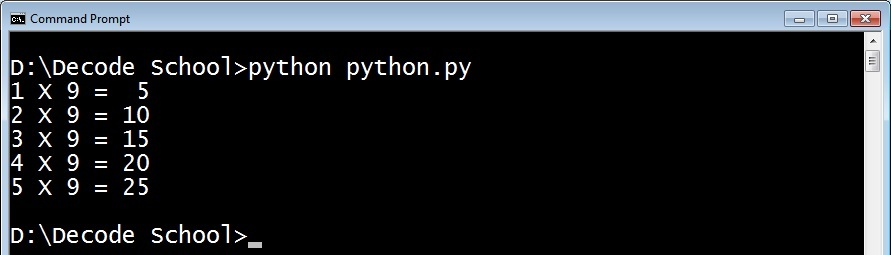Print Multiplication table in with Alignment

you can see that answers in the table are right aligned by format() method.

### Billing Information

``````print("{:20} {:^5} {:>5} {:>6}".format('Product', 'Price', 'Qty', 'Amount' ))
print("{:20} {:>5} {:>5} {:>6}".format('Hard Disk', '200', '1', '200' ))
print("{:20} {:>5} {:>5} {:>6}".format('Keyboard', '150','10', '1500' ))
print("{:20} {:>5} {:>5} {:>6}".format('Mouse','100', '2', '200' ))
print("{:20} {:>5} {:>5} {:>6}".format('Total', '','', '1900' ))``````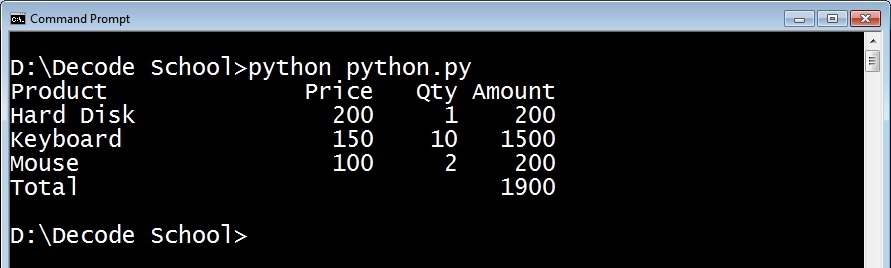Here also, Products are properly left aligned and others are right aligned.

We have learned string output formatting in this article, and we will learn the formatting of numbers and input formatting in coming posts.